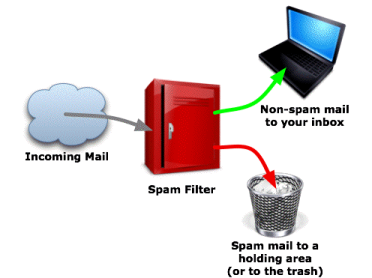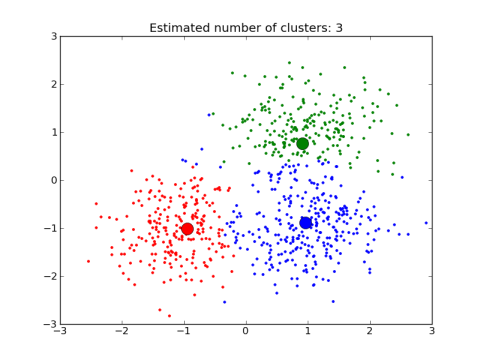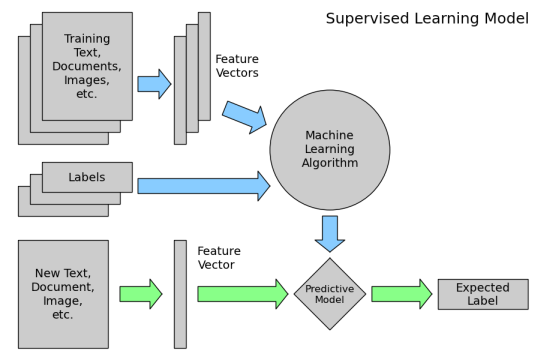An easy introduction to machine learning
An easy introduction to machine learning

Topics¶

1. What is machine learning?
2. What are the two main categories of machine learning?
3. How does machine learning work for supervised learning (predictive modelling)?
4. Big questions in learning machine learning
5. Resources

This tutorial is derived from Data School's Machine Learning with scikit-learn tutorial. I added my own notes so anyone, including myself, can refer to this tutorial without watching the videos.

1. What is machine learning?¶

High level definition: semi-automated extraction of knowledge from data

• Starts with data: You need data to exact insights from it
• Knowledge from data: Starts with a question that might be answerable using data
• Automated extraction: A computer provides the insight
• Semi-automated: Requires many smart decisions by a human

2. What are the two main categories of machine learning?¶

Supervised learning: making predictions using data

• This is also called predictive modelling
• Example: Is a given email "spam" or "ham/non-spam"?
• There is an outcome we are trying to predictUnsupervised learning: extracting structure from data

• Example: Segment grocery store shoppers into clusters that exhibit similar behaviors
• There is no "right answer"3. How does machine learning work for supervised learning (predictive modelling)?¶

2 high-level steps of supervised learning:

1. Train a machine learning model using labeled data

• "Labeled data" has been labeled with the outcome
• "Machine learning model" learns the relationship between the attributes of the data and its outcome
2. Make predictions on new data for which the label is unknownThe primary goal of supervised learning is to build a model that generalizes

• It accurately predicts the future rather than the past

4. Big questions in learning machine learning¶

• How do I choose which attributes of my data to include in the model?
• How do I choose which model to use?
• How do I optimize this model for best performance?
• How do I ensure that I'm building a model that will generalize to unseen data?
• Can I estimate how well my model is likely to perform on unseen data?

Tags: